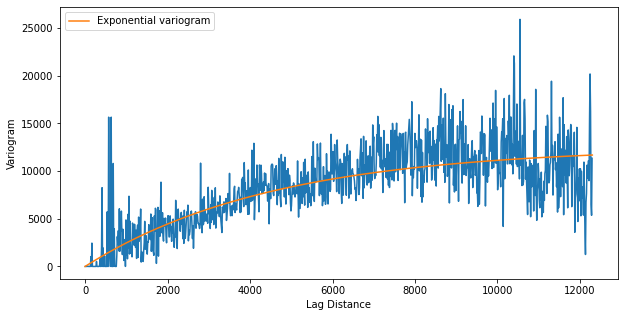# 2.2-kriging

%matplotlib inline
from pyvista import set_plot_theme
set_plot_theme('document')

# Kriging with GSTools¶

This example utilizes data available from the FORGE geothermal reserach site <https://utahforge.com>'s 2019 Geothermal Design Challenge <https://utahforge.com/studentcomp/>. In this example, the data is archived in the Open Mining Format v1 (OMF) <https://github.com/gmggroup/omf>_ specification and the omfvista <https://opengeovis.github.io/omfvista/>_ software is leverage to load those data into a PyVista MultiBlock data structure.

The goal of this workflow is to create a 3D temperature model by kriging the Observed Temperature data (sparse observational data). The open-source, Python software GSTools <https://geostat-framework.github.io/>_ is used to perform variogram analysis and kriging of the temperature data onto a PyVista mesh to create the 3D model.

# sphinx_gallery_thumbnail_number = 4
import pyvista as pv
from pyvista import examples
import numpy as np
import omfvista
import PVGeo
import matplotlib.pyplot as plt
from gstools import Exponential, krige, vario_estimate_unstructured
from gstools.covmodel.plot import plot_variogram

For this project, we have two data archives in the Open Mining Format (OMF) <https://github.com/gmggroup/omf>_ specification and we will use one of PyVista's companion projects, omfvista <https://opengeovis.github.io/omfvista/>_ to load those data archives into PyVista a MultiBlock dataset.

url = "https://dl.dropbox.com/s/3cuxvurj8zubchb/FORGE.omf?dl=0"

project

## Initial Inspection¶

Now we can go ahead and create an integrated visualization of all of the data available to us.

p = pv.Plotter(window_size=np.array([1024, 768]) * 2)
project["Site Boundary"], color="yellow", render_lines_as_tubes=True, line_width=10
)
project["Observed Temperature"],
cmap="coolwarm",
clim=[10, 270],
point_size=15,
render_points_as_spheres=True,
)

p.enable_depth_peeling()
p.camera_position = [
(315661.9406719345, 4234675.528454831, 15167.291249498076),
(337498.00521202036, 4260818.504034578, -1261.5688408692681),
(0.2708862567924439, 0.3397398234107863, 0.9006650255615491),
]
p.show()

## Kriging¶

Now we will use an external library, gstools, to krige the temperature probe data into a 3D temperature model of the subsurface.

### Choosing a Model Space¶

We start out by creating the 3D model space as a PyVista UniformGrid that encompasses the project region. The following were chosen manually:

# Create the kriging grid
grid = pv.UniformGrid()
# Bottom south-west corner
grid.origin = (329700, 4252600, -2700)
# Cell sizes
grid.spacing = (250, 250, 50)
# Number of cells in eaxh direction
grid.dimensions = (60, 75, 100)

Visually inspect the kriging grid in relation to data

p = pv.Plotter(window_size=np.array([1024, 768]) * 2)
project["Observed Temperature"],
cmap="coolwarm",
stitle="Temperature (C)",
point_size=15,
render_points_as_spheres=True,
)
p.enable_depth_peeling()
p.camera_position = [
(303509.4197523619, 4279629.689766085, 8053.049483835099),
(336316.405356571, 4261479.748583805, -1756.358124546427),
(0.22299463811939535, -0.11978828465250713, 0.9674317331109259),
]
p.show()

### Variogram Analysis¶

Next, we need to compute a variogram for the temperature probe data and fit that variogram to an exponential model

bins = np.linspace(0, 12300, 1000)
bin_center, gamma = vario_estimate_unstructured(
project["Observed Temperature"].points.T,
project["Observed Temperature"]["temperature (C)"],
bins,
)

fit_model = Exponential(dim=3)
fit_model.fit_variogram(bin_center, gamma, nugget=False)

plt.figure(figsize=(10, 5))
plt.plot(bin_center, gamma)
plot_variogram(fit_model, x_max=bins[-1], ax=plt.gca())
plt.xlabel("Lag Distance")
plt.ylabel("Variogram")
plt.show()
/opt/homebrew/Caskroom/miniforge/base/lib/python3.9/site-packages/gstools/covmodel/plot.py:112: UserWarning: Matplotlib is currently using module://matplotlib_inline.backend_inline, which is a non-GUI backend, so cannot show the figure.
fig.show()### Perfroming the Kriging¶

Then we pass the fitted exponential model when instantiating the kriging operator from GSTools.

# Create the kriging model
krig = krige.Ordinary(
fit_model,
project["Observed Temperature"].points.T,
project["Observed Temperature"]["temperature (C)"],
)

After instantiating the kriging operator, we can have it operate on the nodes of our 3D grid that we created earlier and collect the results back onto the grid.

krig.mesh(
grid,
name="temperature (C)",
)
(array([116.47318011, 117.61463724, 118.68608621, ..., 38.14076571, 39.54938107, 40.95250078]), array([8097.97043138, 7671.53933859, 7233.85868221, ..., 8755.23073853, 9108.5391645 , 9445.15771335]))

And now the grid model has the temperature scalar field and kriging variance as data arrays.

project["Kriged Temperature Model"] = grid

## Post-Processing¶

We can use a filter from PVGeo to extract the region of the temperature model that is beneath the topography surface as the kriging did not account for the surface boundary.

# Instantiate the algorithm
extractor = PVGeo.grids.ExtractTopography(tolerance=10, remove=True)
# Apply the algorithm to the PyVista grid using the topography surface
subsurface = extractor.apply(grid, project["Terrain"])

## Resulting Visualization¶

# Create the main figure
"""A helper to add data to scenes."""
project["Site Boundary"].tube(50),
color="yellow",
)

subsurface.ctp().contour([175, 225]),
name="the model",
scalars="temperature (C)",
cmap="coolwarm",
clim=[10, 270],
opacity=0.9,
stitle="Temperature (C)",
)
project["Observed Temperature"],
cmap="coolwarm",
clim=[10, 270],
render_points_as_spheres=True,
point_size=10,
stitle="Temperature (C)",
)

p.enable_depth_peeling(20)
return

p = pv.Plotter()

p.camera_position = [
(319663.46494887985, 4294870.4704494225, -8787.973684799075),
(336926.4650625, 4261892.29012, 103.0),
(-0.09983848586767283, 0.20995262898057362, 0.9726007250273854),
]

p.show()
/opt/homebrew/Caskroom/miniforge/base/lib/python3.9/site-packages/pyvista/plotting/plotting.py:2052: PyvistaDeprecationWarning:
"stitle" is a depreciated keyword and will be removed in a future
release.

Use scalar_bar_args instead.  For example:

scalar_bar_args={'title': 'Scalar Bar Title'}


---------------------------------------------------------------------------
ValueError                                Traceback (most recent call last)
Input In , in <cell line: 37>()
32     return
35 p = pv.Plotter()
39 p.camera_position = [
40     (319663.46494887985, 4294870.4704494225, -8787.973684799075),
41     (336926.4650625, 4261892.29012, 103.0),
42     (-0.09983848586767283, 0.20995262898057362, 0.9726007250273854),
43 ]
45 p.show()

10 p.add_mesh(project["Opal Mound Fault"], color="brown", opacity=0.75)
11 p.add_mesh(project["Negro Mag Fault"], color="lightblue", opacity=0.75)
14     subsurface.ctp().contour([175, 225]),
15     name="the model",
16     scalars="temperature (C)",
17     cmap="coolwarm",
18     clim=[10, 270],
19     opacity=0.9,
20     stitle="Temperature (C)",
21 )
23     project["Observed Temperature"],
24     cmap="coolwarm",
(...)
28     stitle="Temperature (C)",
29 )
31 p.enable_depth_peeling(20)

File /opt/homebrew/Caskroom/miniforge/base/lib/python3.9/site-packages/pyvista/plotting/plotting.py:2183, in BasePlotter.add_mesh(self, mesh, color, style, scalars, clim, show_edges, edge_color, point_size, line_width, opacity, flip_scalars, lighting, n_colors, interpolate_before_map, cmap, label, reset_camera, scalar_bar_args, show_scalar_bar, multi_colors, name, texture, render_points_as_spheres, render_lines_as_tubes, smooth_shading, split_sharp_edges, ambient, diffuse, specular, specular_power, nan_color, nan_opacity, culling, rgb, categories, silhouette, use_transparency, below_color, above_color, annotations, pickable, preference, log_scale, pbr, metallic, roughness, render, component, **kwargs)
ValueError: Empty meshes cannot be plotted. Input mesh has zero points.